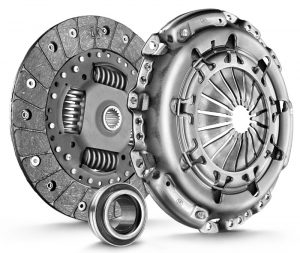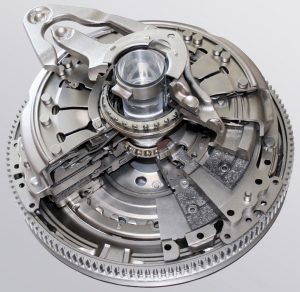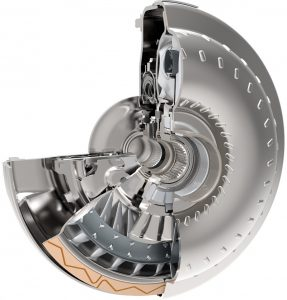# How to calculate the torque capacity of a clutch

Modern automotive powertrain and drivetrain systems have at least one clutch as a component. An AWD or 4WD vehicle can have several clutches, depending on the architecture and type of the powertrain and drivetrain.

A clutch can be a standalone component, used to connect the internal combustion engine (ICE) to the transmission, or can be the sub-component or another main component: torque converter, automatic transmission, transfer case, limited-slip differential (LSD), etc.

Depending on the number of friction plates, a clutch can be:

• single plate clutch
• multi-disc clutch

Depending on the type of friction, we can have:

• dry clutches
• wet (oil) clutches

Usually, single plate clutches (except torque converter lock-up clutches) have dry friction and multi-disc clutches are with wet friction.Image: Single-plate dry clutch (LuK)Credit: LuKImage: Dry double-clutch (LuK)Credit: LuKImage: Torque converter with lock-up clutch (LuK)Credit: LuKImage: Multi-disc wet double clutch (DualTronic – BorgWarner)Credit: BorgWarner

In operation, a clutch can have 4 operating states:

• open, zero torque is transmitted between the input and the output shafts
• slipping, some amount of torque is transmitted between input and output shafts; the speed difference between input and output shaft is significant (e.g. 500 rpm)
• micro-slipping, almost all of the input torque is transmitted through the clutch; the speed difference between input and output shaft is very small, around 5-10 rpm
• closed (clamped, locked-up), there is no slip between input and output shaft, all the input torque is transmitted through the clutch

Regardless of the type, every clutch has a torque capacity. The torque capacity of the clutch is the amount of torque that can be transmitted by the clutch when it’s slipping or when it’s fully closed. The torque capacity of a clutch depends on a series of factors:

• total area of the friction surface
• friction coefficient
• normal force acting on the clutch
• number of friction elements

To calculate the torque capacity of the clutch we’ll have a look at the geometry of the clutch (friction) disc. Within the area of the friction surface we are going to represent an elementary area dx, at the distance x from the center.

where:

Fa [N] – the normal force pressing the clutch plate
Tc [Nm] – the torque capacity of the clutch
r1 [m] – the inner radius of the friction surface
r2 [m] – the outter radius of the friction surface

The pressure p [Pa] acting on the clutch is equal with the ratio between the normal force Fa and the area of the friction surface S [m2]:

$p = \frac{F_a}{S} \tag{1}$

Assuming that the area of the rivets is neglijable, the area of the friction surface is calculated as:

$S = S_2 – S_1 = \pi r_2^2 – \pi r_1^2 = \pi (r_2^2 – r_1^2) \tag{2}$

Replacing (2) in (1), we get the expression for the clutch pressure:

$p = \frac{F_a}{\pi (r_2^2 – r_1^2)} \tag{3}$

The elementary area dA is calculated as:

$dA = 2 \pi x dx \tag{4}$

The elementary normal force dN, acting on the elementary area is calculated as:

$dN = p dA \tag{5}$

Replacing (3) and (4) in (5), we get:

$dN = \frac{2 F_a x dx}{r_2^2 – r_1^2} \tag{6}$

The elementary friction force dF is calculated as:

$dF = \mu dN \tag{7}$

where μ [-] is the friction coefficient of the clutch disc.

Replacing (6) in (7), we get:

$dF = \frac{2 \mu F_a x dx}{r_2^2 – r_1^2} \tag{8}$

The elementary friction torque dT is calculated as:

$dT = x dF \tag{9}$

Replacing (8) in (9), we get:

$dT =\frac{2 \mu F_a x^2 dx}{r_2^2 – r_1^2} \tag{10}$

Integrating equation (10) from r1 to r2, we get the mathematical expression of the torque capacity of the clutch:

$\begin{equation*} \begin{split} T_c &= \int_{r_1}^{r_2} dT & \\ &= \frac{2 \mu F_a}{r_1^2 – r_2^2} \int_{r_1}^{r_2} x^2 dx \\ &= \frac{2}{3} \mu \frac{r_2^3 – r_1^3}{r_2^2 – r_1^2} F_a \end{split} \end{equation*}$

Since a clutch has two friction surfaces, the resulting mathematical expression for the torque capacity of a single plate clutch is:

$\bbox[#FFFF9D]{T_c = 2 \cdot \frac{2}{3} \mu \frac{r_2^3 – r_1^3}{r_2^2 – r_1^2} F_a} \tag{11}$

For a multi-disc clutch expression (11) becomes:

$\bbox[#FFFF9D]{T_c = z \frac{2}{3} \mu \frac{r_2^3 – r_1^3}{r_2^2 – r_1^2} F_a} \tag{12}$

where z [-] is the number of friction surfaces (e.g. 2 – for a single friction disc, 4 – for two friction discs, etc.).

Assuming a mean radius rm [m] of the clutch calculated as:

$r_m = \frac{r_2 + r_1}{2}$

we can deduce a simplified expression, with an acceptable error, for the torque capacity of the clutch:

$T_c = z \mu F_a r_m$

Example 1. Calculate the torque capacity of single plate dry clutch, which has: the normal force 250 N, the outer radius 0.3 m, the inner radius 0.2 m and the friction coefficient 0.4.

Replacing the given parameters in equation (11), we get:

$T_c = 2 \cdot \frac{2}{3} \cdot 0.4 \cdot \frac{0.3^3 – 0.2^3}{0.3^2 – 0.2^2} \cdot 250 = 50.7 \text{ Nm}$

Example 2. Calculate the torque capacity of multi-disc wet clutch, which has: 5 friction discs (plates), the normal force 250 N, the outer radius 0.3 m, the inner radius 0.2 m and the friction coefficient 0.07.

Replacing the given parameters in equation (12), we get:

$T_c = 10 \cdot \frac{2}{3} \cdot 0.07 \cdot \frac{0.3^3 – 0.2^3}{0.3^2 – 0.2^2} \cdot 250 = 44.3 \text{ Nm}$

The same algorithm can be used to calculate the braking torque of a vehicle equipped with disc brake system.

You can also check your results using the calculator below.

### Clutch Torque Calculator

 z [-] μ [-] r2 [m] r1 [m] Fa [N] Clutch Torque, Tc [Nm] =

For any questions, observations and queries regarding this article, use the comment form below.

Don’t forget to Like, Share and Subscribe!